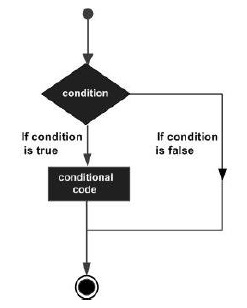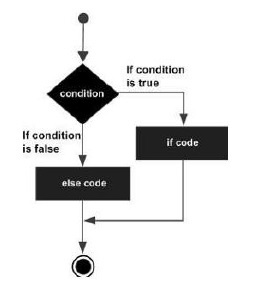# Rust - Decision Making

Decision-making structures require that the programmer specify one or more conditions to be evaluated or tested by the program, along with a statement or statements to be executed if the condition is determined to be true, and optionally, other statements to be executed if the condition is determined to be false.

Shown below is the general form of a typical decision-making structure found in most of the programming languages −Sr.No Statement & Description
1

if statement

An if statement consists of a Boolean expression followed by one or more statements.

2

if...else statement

An if statement can be followed by an optional else statement, which executes when the Boolean expression is false.

3

else...if and nested ifstatement

You can use one if or else if statement inside another if or else if statement(s).

4

match statement

A match statement allows a variable to be tested against a list of values.

## If Statement

The if…else construct evaluates a condition before a block of code is executed.

### Syntax

```if boolean_expression {
// statement(s) will execute if the boolean expression is true
}
```

If the Boolean expression evaluates to true, then the block of code inside the if statement will be executed. If the Boolean expression evaluates to false, then the first set of code after the end of the if statement (after the closing curly brace) will be executed.

```fn main(){
let num:i32 = 5;
if num > 0 {
println!("number is positive") ;
}
}
```

The above example will print number is positive as the condition specified by the if block is true.

## if else statement

An if can be followed by an optional else block. The else block will execute if the Boolean expression tested by the if statement evaluates to false.

### Syntax

```if boolean_expression {
// statement(s) will execute if the boolean expression is true
} else {
// statement(s) will execute if the boolean expression is false
}
```

### FlowChartThe if block guards the conditional expression. The block associated with the if statement is executed if the Boolean expression evaluates to true.

The if block may be followed by an optional else statement. The instruction block associated with the else block is executed if the expression evaluates to false.

### Illustration - Simple if…else

```fn main() {
let num = 12;
if num % 2==0 {
println!("Even");
} else {
println!("Odd");
}
}
```

The above example prints whether the value in a variable is even or odd. The if block checks the divisibility of the value by 2 to determine the same. Here is the output of the above code −

```Even
```

## Nested If

The else…if ladder is useful to test multiple conditions. The syntax is as shown below −

### Syntax

```if boolean_expression1 {
//statements if the expression1 evaluates to true
} else if boolean_expression2 {
//statements if the expression2 evaluates to true
} else {
//statements if both expression1 and expression2 result to false
}
```

When using if…else…if and else statements, there are a few points to keep in mind.

• An if can have zero or one else's and it must come after any else..if.
• An if can have zero to many else..if and they must come before the else.
• Once an else..if succeeds, none of the remaining else..if or else will be tested.

```fn main() {
let num = 2 ;
if num > 0 {
println!("{} is positive",num);
} else if num < 0 {
println!("{} is negative",num);
} else {
println!("{} is neither positive nor negative",num) ;
}
}
```

The snippet displays whether the value is positive, negative or zero.

### Output

```2 is positive
```

## Match Statement

The match statement checks if a current value is matching from a list of values, this is very much similar to the switch statement in C language. In the first place, notice that the expression following the match keyword does not have to be enclosed in parentheses.

The syntax is as shown below.

```let expressionResult = match variable_expression {
constant_expr1 => {
//statements;
},
constant_expr2 => {
//statements;
},
_ => {
//default
}
};
```

In the example given below, state_code is matched with a list of values MH, KL, KA, GA − if any match is found, a string value is returned to variable state. If no match is found, the default case _ matches and value Unkown is returned.

```fn main(){
let state_code = "MH";
let state = match state_code {
"MH" => {println!("Found match for MH"); "Maharashtra"},
"KL" => "Kerala",
"GA" => "Goa",
_ => "Unknown"
};
println!("State name is {}",state);
}
```

### Output

```Found match for MH
State name is Maharashtra
```